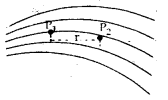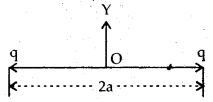# MCQ Questions for Class 12 Physics Chapter 1 Electric Charges and Fields with Answers

We have compiled the NCERT MCQ Questions for Class 12 Physics Chapter 1 Electric Charges and Fields with Answers Pdf free download covering the entire syllabus. Practice MCQ Questions for Class 12 Physics with Answers on a daily basis and score well in exams. Refer to the Electric Charges and Fields Class 12 MCQs Questions with Answers here along with a detailed explanation.

## Electric Charges and Fields Class 12 MCQs Questions with Answers

Question 1.
In comparison With the electrostatic force between two electrons, the electrostatic force between two protons is:
(a) greater
(b) smaller
(c) zero
(d) same.

Question 2.
If electric flux through a closed surface is zero. It means that:
(a) the net charge inside the surface is zero.
(b) the electric field is necessarily zero at all points on the surface.
(c) no charge exists inside the surface.
(d) no charge exists outside the surface.

Answer: (a) the net charge inside the surface is zero.

Question 3.
Static electricity is produced by
(a) induction only
(b) friction
(c) chemical reaction only
(d) friction and induction both.

Question 4.
When distance between two charges is reduced to one-half of the original distance, the force between them will remain the same if one of the charges is made:
(a) one fourth
(b) four times
(c) double
(d) one-halftimes.

Question 5.
A unit Coulomb charge is one which when placed in air at a distance of 1 m from an equal and similar charge repel it with a force of
(a) 9 × 109 N
(b) 1 N
(c) 1 dyne
(d) None of these.

Answer: (a) 9 × 109 N

Question 6.
When two charged spheres are connected with a wire, the electric charge on them is shared:
(a) inversely as their capacity
(b) equally
(c) in proportional to their capacity
(d) None of these.

Question 7.
An electric dipole of moment p is placed in the position of stable equilibrium in a uniform electric field $$\vec{E}$$. The couple required to rotate it through an angle 6 the initial position is:
(a) -PE cos θ
(b) PE tan θ
(c) PE cos θ
(d) PE sin θ.

Question 8.
Two point charges each of 20 µC are placed 50 cm apart in air. What is the electric field intensity at the mid point on the line joining the centre of two point charges?
(a) 5 × 106 NC-1
(b) 18 × 106 NC-1
(c) Zero
(d) None of these

Question 9.
Two charges 10 pC and 5 pC are placed 20 cm apart. The ratio of Coulomb’s force experienced by there is:
(a) 2 : 5
(b) 1 : 1
(c) √3 = √7
(d) None of these

Question 10.
The figure here shows electric field lines. The electric field strength at P1 is E1 and that at P2 is E2 If distance between P1, P2 is r, then which of the following statement is true?(a) E1 > E2
(b) E1 < E2
(c) E2 = rE1
(d) E2 = E1/r²

Question 11.
The surface charge density oil the copper sphere is σ. The electric field strength on the surface of Sphere of radius r is:
(a) $$\frac {σ}{2}$$
(b) σ
(c) $$\frac {σ}{2ε_0}$$
(d) σ/ε0

Question 12.
A sphere ‘P’ of mass 6.4 × 10-15 kg is hanging between the two charged parallel plates as shown in figure. How many electrons can be added or removed from the sphere so that it still remains hanging?(a) 8 electrons can be added
(b) 8 electrons can be removed
(c) No. electron can be added or removed
(d) 8 electrons can be either removed or added.

Answer: (b) 8 electrons can be removed

Question 13.
Which of the following method is an indirect method of charging:
(a) charging by induction
(b) charging by physical contact
(c) charging by friction
(d) none of these.

Question 14.
An electron and a proton are placed in the same uniform electric field. What will be the ratio of the acceleration of electron to that of proton?
(a) 1
(b) zero
(c) $$\frac {m_p}{m_e}$$
(d) $$\frac {m_e}{m_p}$$

Answer: (c) $$\frac {m_p}{m_e}$$

Question 15.
The position of the charge inside the enclosing surface is changed in such a way that the total charge remains constant. Then the total normal electric flux through the enclosing surface:
(a) increases
(b) decreases
(c) changes erratically
(d) Remains unchanged.

Fill in the Blanks

Question 1.
The force between two point charges ………………… when dielectric constant of the medium in which they are held increases.

Question 2.
When a dielectric medium is placed in a uniform electric field, the dielectric constant of the medium is the ratio of the ……………….. of the medium to the ……………… of free space.

Question 3.
Two identical charges are placed at a distance 2a apart as shown in figure. The potential energy of the positively charged particle placed at the mid point O will be …………….Question 4.
The number of electric lines of force that radiate outward from 1C charge is ………………